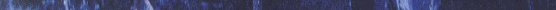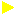# INSERTION SORT## An efficient algorithm for sorting a small number of elements.Though it has a worst cae running time of O(n^2),it is in general much faster in two cases : (i) The small constant factors in insertion sort make it faster for small n. (ii) It is faster when parts of input list are already sorted. After k passes(where each pass requires at the most scanning all elements of the list)the first k elements of the list are sorted.Since each pass has a worst case running time of O(n) and there are total of n passes,therefore the worst case running time of insertion sort is O(n*n)ANIMATIONTHE ALGORITHMApplet page